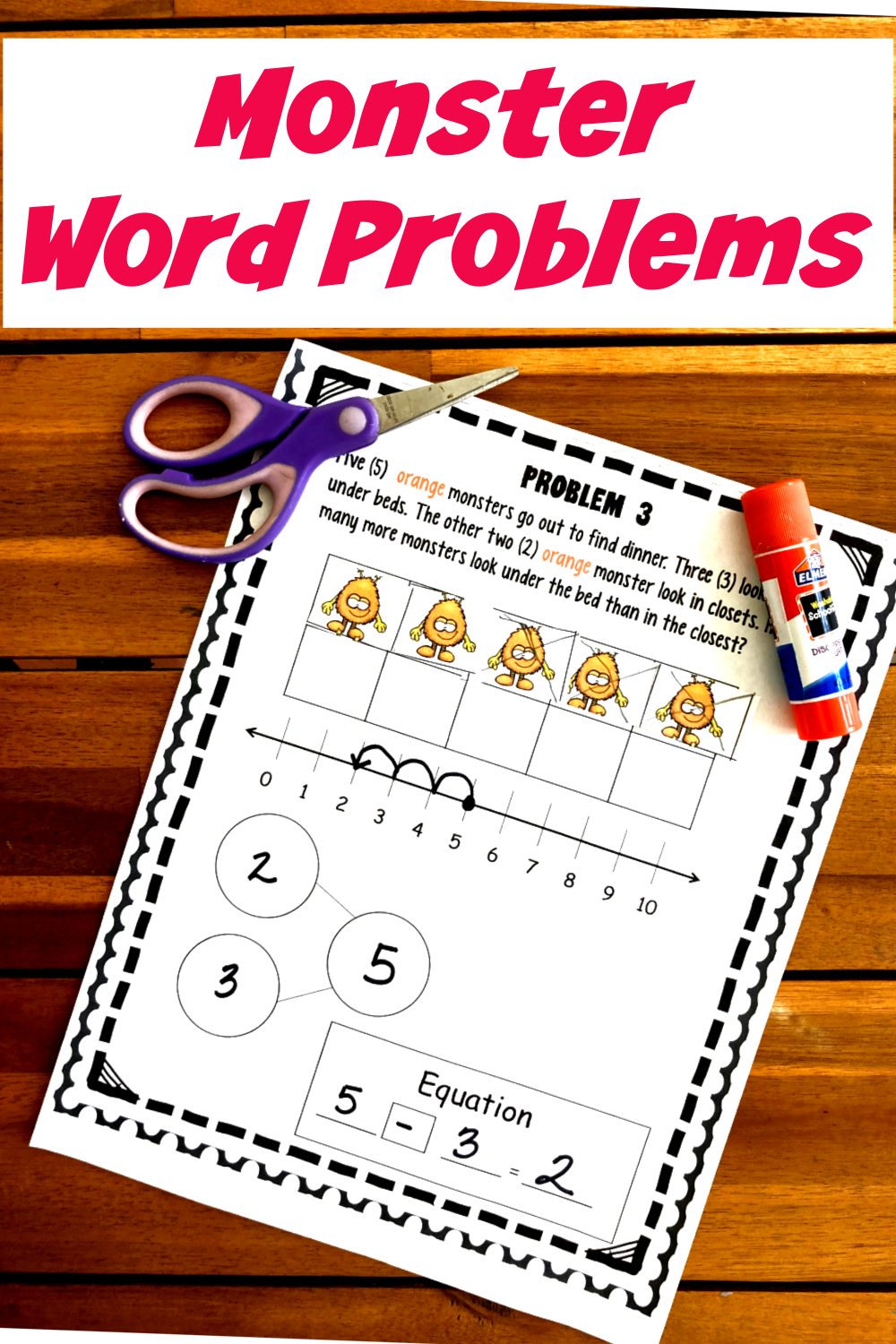# Monster-themed Word Problems within 10 | Free Printable

## Word Problems within 10

Need to work on solving word problems within 10? Check out these 6 FREE monster word problems with ten grids, number lines, number bonds, and equations.

I always dreaded it. I had finally finished the 20 problems and was sick and tired of computing. But I wasn’t done.

I was now required to solve those nasty word problems glaring at me from the bottom of the page. They were intimidating and one more step that was required of me.

My only hope was that the needed operation was the same one I had been doing for the past 30 minutes.

I definitely don’t want that type of math experience for my students or children. With that thought, I’ve created word problems that are interactive and aren’t done after tediously working through multiple math problems.

This fun monster word problem set has five steps and is designed to build number sense as well as knowledge of how to solve word problems.

### Step 1: Word Problem Vocabulary

If your students are new to word problems, I highly suggest that you start with this activity from This Reading Mama. It helps children learn the math word problem vocabulary most often found in word problems, and what to do with it.

Once they have completed that activity, they are ready for the monster math word problems. Simply have them read the word problem… or read it to them… and have them underline the special vocabulary words they find.

Discuss with your little ones what they mean, and what operations need to be done.

### Step 2: Ten Frames Word Problems

On each word problem, a ten frame is included and is probably the easiest part of the page. The students simply cut out the monsters that match the word problem and glue the correct amount into the ten frames.

There are some comparison subtraction problems included, and we found it was easiest to put one set of monsters on the top row and the second set of monsters on the second row.

This way my kindergartener could easily see how many more monster was in one of the sets.

### Step 3: Number Line Word Problems

The goal of having children using a number line is not to get the right answers. According to Think Math, using the number line can improve mental computation, increase an understanding of what the answers mean, and even lead to a better understanding of negative numbers.

It also increases their understanding of addition and subtraction. Students must know whether to move up the number line or down the number line based on the word problem.

After doing a few of the monster word problems, my kindergartner quickly picked up placing a dot at the starting point and then moving up or down the number line to the answer to the problem.

### Step 4: Number Bonds Word Problems

Marnel Infant School says, “Knowing addition number bonds means that your child will understand subtraction with ease. If they know that 3+4 = 7 they will quickly realize that when they see 7 – 4 = ? the answer is 3 because by a process of elimination 3 is the missing number in this number bond. It helps children to see that subtraction is a mirror image of addition.”

After completing the ten grid and the number line, students must fill in the number bond with the numbers from the word problem.

Get extra number bond practice with these fun monster task cards.

### Step 5: Equation Word Problems

After all the math your students have done, this last part is easy. All they need to do is look at the number bonds and write an equation that best illustrates what they did to solve their monster word problem.

If you have been doing any type of addition or subtraction with children, most of them can correctly write an addition problem… especially when the number bonds are already on the paper.

Even those children that have been exposed to addition and subtraction problems, often struggle to create correct subtraction number sentences.

You will need to teach your precious little ones that the number you started with goes first, then what you subtracted from goes second, and then what is left is what goes into the equal part.

Once you and your kiddos have worked through these five steps numerous times, they will have a strong foundation for solving other subtraction and addition word problems they encounter.

So if you are ready to have some fun with word problems and build number sense in the process, enjoy this free printable.

## Addition and Subtraction Word Problems Worksheets

Below are the free monster addition and subtraction word problems in 10 worksheets. You can download these worksheets by clicking on the download button.

word-monster-word-problems-3

Get more word problems HERE.

Want to keep the monster theme? Try some of these monster logic problems printables.